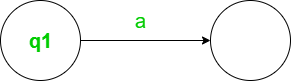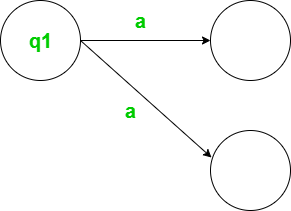GFG App
Open AppBrowser
Continue

# Difference Between NPDA and DPDA

A Pushdown Automata (PDA) is a way to implement context-free Grammar in a similar way. We design Finite Automata for Regular Grammar.

1. It is more powerful than FSM.
2. FSM has very limited memory but PDA has more memory.
3. PDA= Finite State Machine + Stack

This stack has infinite memory and that facilitates the higher power of Pushdown automata. This helps PDA to behave more powerful than Finite-state Machine.

### A Pushdown Automata has three Components:

1. An input tape
2. A finite Control Unit.
3. A stack with infinite size.

#### Definition of DPDA and NPDA with example

Let M = (Q,∑,Γ,q0, Z,F ,δ) be a PDA. The PDA is deterministic if and only if

1. δ(q, a,  Z) has one element.
2. If δ(q, ∈,  Z) is non-empty, then δ(q, c,  Z) should be empty for every c ∈ ∑ .

Both conditions should be satisfied for the PDA to be deterministic. If one of the conditions fails, the PDA is non-deterministic.

Let us consider an example to understand more clearly.

Example: Is the PDA corresponding to the language L = {anbn | n>=1} by the finite state is deterministic ?

Solution: The shown transitions defined for this machine are shown below:

```δ(q0, a,  Z0) =δ(q0, aZ0)
δ(q0, a,  a) =δ(q0, aa)
δ(q0, b,  a) =δ(q1, ∈)
δ(q1, b,  a) =δ(q1, ∈)
δ(q1, ∈,  Z0) =δ(qf, ∈) ```

The PDA should satisfy the two conditions. shown in the definition to be deterministic.

1. δ(q0, a,  Z0) has only one element. In this case, for each q∈Q, a∈∑ , and Z∈Γ, there exists only one definition so the first condition is satisfied.
2. To satisfy the second condition consider the transition. δ(q1,∈ ,  Z0) = (qf,∈,). Since the transition is defined, the transition δ(q, a,  Z0) where a∈∑ should not be defined which is true.

since both conditions. are satisfied, then the given PDA is deterministic.

### Difference between NPDA and DPDA:

#### There is one more difference as follows:

DPDA: For every input with the current state, there is only one move.

M = (Q,∑,Γ,q0, Z,F ,δ)

δ: Q*∑*Γ→Q*ΓDeterministic path

NPDA: For every input with the current state, We can have multiple moves.

M = (Q,∑,Γ,q0, Z,F ,δ)

δ: Q*{∑∪∈}*Γ→2Q*Non- Deterministic path

### Why NPDA is more powerful than DPDA?

NDPA is more powerful than DPDA because we can add more transitions to it. It is possible for every language to add a transition. For some languages, we can construct DPDA there exist an NPDA but there are some languages that are accepted by NPDA but are not by DPDA. This is said to be powerful when it accepts more sets of languages than other automata.

In fact, it is more powerful than DFA(Deterministic finite automata) and NFA(Non-deterministic finite automata) also because, In the case of DFA and NFA, they are equivalent in power. So for every language accepted by DFA there exist an NFA and Vice-Versa. There is not any language for which we construct NFA but not DFA. Hence, we can’t convert NPDA to DPDA always and we can convert NFA to equivalent DFA always.